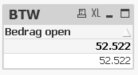# QlikView App Dev

Discussion Board for collaboration related to QlikView App Development.

Announcements
Now Live: Qlik Sense SaaS Simplified Authoring – Analytics Creation for Everyone: READ DETAILS
cancel
Showing results for
Did you mean:Contributor III

## Unknown error at expression while the same expression works correctly in dashboard

In my dashboard I have the following expression:

NUM(SUM({\$<Boekjaar = {\$(=MAX(Boekjaar))}, PeriodeId = {"<=\$(=MAX({<Boekjaar={\$(=MAX(Boekjaar))}>} PeriodeId))"},  BalansOmschrijving1 = {"Overzicht kengetallen"}, BalansOmschrijving3 = {"Tussenrekeningen voor BTW"} >} Saldo), '###.###.###.##0')

where it gives the correct value in a straight table.

I need to have the same value in my script but I receive an unknown error when executing the script:

Unknown Error
LET vBTW = NUM(NUM(SUM({\$<Boekjaar = {}, PeriodeId = {"<="}, BalansOmschrijving1 = {"Overzicht kengetallen"}, BalansOmschrijving3 = {"Tussenrekeningen voor BTW"} >} Saldo), '###.###.###.##0'), '#,##0')

When I use the same expression in a straight table I get the correct value displayed:Why doesn't work this expression in the script? The variable vBTW needs to be set to 52522 in the script.

The part of the script where the error occurred is:

LET vBTW = NUM(NUM(SUM({\$<Boekjaar = {\$(=MAX(Boekjaar))}, PeriodeId = {"<=\$(=MAX({<Boekjaar={\$(=MAX(Boekjaar))}>} PeriodeId))"},  BalansOmschrijving1 = {"Overzicht kengetallen"}, BalansOmschrijving3 = {"Tussenrekeningen voor BTW"} >} Saldo), '###.###.###.##0'), '#,##0');

Labels (2)

• ### variable

2 RepliesMVP & Luminary

You couldn't calculate in this way within the script else you need to do it within one or maybe several load-statements. Further within the script is no set analysis available and also no adhoc-variable like: \$(= ...).

- MarcusPartner - Contributor III

In the load script you need to use temporary table in order to store such expression value into variable.

```Tmp_table: